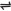## Rules for Writing Equilibrium Constant Expressions

The following are the rules for writing equilibrium constant expressions:

1. Express the given equation as a fraction, such that the numerator is the product of concentration terms of each product raised to a power equal to the stoichiometric coefficient before it in the balanced equation.

The denominator is the product of concentration terms of each reactant raised to a power equal to the stoichiometric coefficient before it in the balanced equation.

2. The constant K equals the value of the fraction

Example: Write equilibrium constant expressions for the following reversible reactions:

(1).

 CO(g) + NO2(g)CO2(g) + NO(g)

Solution:

Product of concentration terms of products - [CO2][NO]

Product of concentration terms of reactants - [CO][NO2]

Therefore, equilibrium constant Kc = [CO2][NO] / [CO][NO2]

(2). For the reaction:

 N2(g) + 3H2(g)2NH3(g)

Solution:

Kc = [NH3]2 / [N2] [H2]3

Heterogeneous Equilibrium

This is an equilibrium involving more than one phase. For example, an equilibrium involving solid and gaseous phases; liquid and gaseous phases.

In setting up equilibrium constant expressions for heterogeneous equilibriums, we must ignore any term that represents the concentration or partial pressure of a pure solid or liquid.

This is because the concentration of a pure solid or liquid substance is proportional to its density, and therefore remains constant so long as its temperature is not changed.

Equilibrium constant expressions show only gaseous and dissolved species involved in the equilibrium.

Example: (1). For the reaction:

 CaCO3(s)CaO(s) + CO2(g)

Kc = [CO2(g)]

Note: since the concentrations of CaO and CaCO3 are of pure solids, they are therefore ignored. This then means that the equilibrium position of the reaction is determined only by the concentration or partial pressure of CO2(g).

Hence, increasing or decreasing the concentrations of CaCO3 or CaO will not change the equilibrium position. There is only one pressure at which CO2 gas can be in equilibrium with solid CaCO3 and solid CaO.

At 900oC, this pressure is 790 mmHg. If solid CaCO3 and solid CaO are present in the same container where the temperature is 900oC, three possibilities exist:

(i). If CO2 is present at a pressure of 790 mmHg, equilibrium prevails and there will be no change in the amount of CaCO3 and CaO in the system;

(ii). If CO2 is present at a pressure in excess of 790 mmHg, the reaction will proceed to the left, producing more CaCO3 and consuming CaO and CO2 until the quantity of CO2 consumed is sufficient to reduce the pressure of the remaining CO2 to 790 mmHg.

(iii). If CO2 is present at a pressure less than 790 mmHg, or if no CO2 is present, the reaction will proceed to the right, consuming CaCO3 and producing CaO and CO2 until a sufficient quantity of CO2 is present to exert a pressure of 790 mmHg.

(2). For the reaction:

 CuO(s) + H2(g)Cu(s) + H2O(g)

Kc = [H2O(g)] / [H2(g)]

Equilibrium position for the above reaction is dependent only on the concentrations of H2O(g) and H2(g).

Introducing more H2(g) gas into the system that is already in equilibrium will shift the equilibrium towards the right, increasing the amount of gaseous H2O present, until the equilibrium ratio of [H2O(g)] to [H2(g)] is restored.

On the other hand, the introduction of more solid copper oxide into the system will have no effect on the equilibrium.

(3). A solid or liquid substance in equilibrium with its vapor: example,

 I2(s)I2(g)

Kc = [I2(g)]

 H2O(l)H2O(g)

Kc = [H2O(g)]

As long as the temperature is not changed (i.e. constant), a change in the concentration of the solid or liquid will not have any effect on the equilibrium positions of the above reactions.

But an increase in concentration or pressure of the vapor will move the equilibrium backward, while a decrease would move the equilibrium forward.

General Note: Equilibrium constant varies with certain factors, such as the temperatures, pressures and the concentrations of materials in the system. In general, any factor which promotes the forward reaction will increase K, while any factor which favors the backward reaction will decrease K.

The rate law expression for reversible reactions is different from those of direct reactions which has been discussed. For reversible reactions, the exponents on the concentration terms are their coefficients in their balanced equations, while for direct reactions, the exponents are not derived from the balanced equation, but from experiment - these exponents are the order of their reactions.

 Like This Post? Please Share!!!!!!!

Copyright , All Rights Reserved Free Chemistry Online | About Us | Usage of Content | Total Disclosures | Privacy Policy